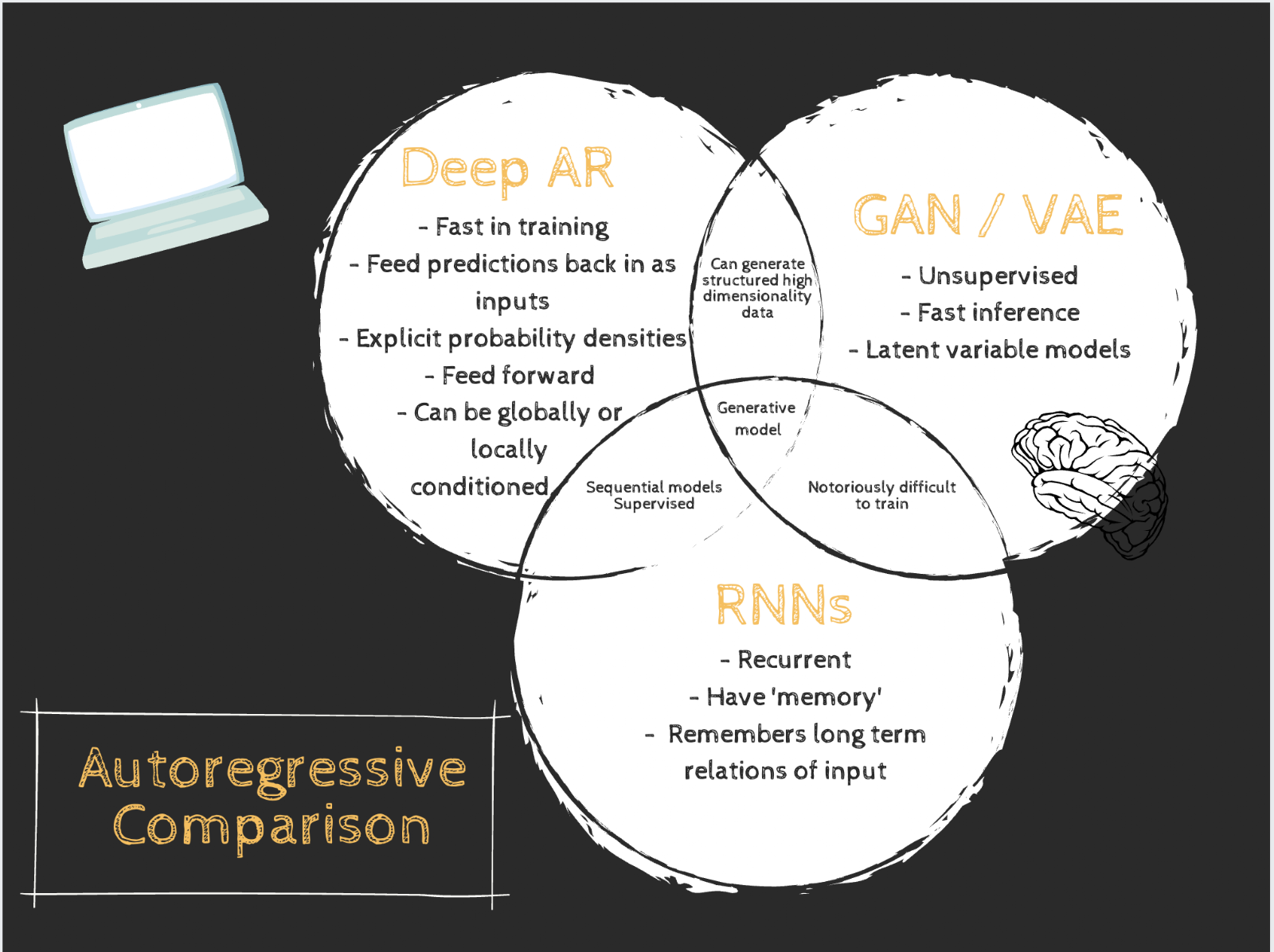# An Intuitive Introduction to Deep Autoregressive Networks

By McClain Thiel

## Introduction

​ Before we dive into deep autoregression, let’s first understand its traditional counterpart. Autoregression simply means regression on self. Autoregression is just predicting a future outcome of a sequence from the previously observed outcomes of that sequence. As an equation, that looks like this: $X_{t+1} = \sum^t_i \delta _i X_{t-i} + c$ . This should look a lot like normal (online) linear regression and that’s because mathematically it effectively is. It uses the previous m terms to predict the next term where m is a constant called the lag or receptive field. The next item, $X_{t+1}$, is predicted based on the product of a learnable parameter, , times a previous item in the sequence, $X_i$, plus a learnable constant or bias, c. The reason this form looks a bit strange is twofold. The first of which is that I want to really highlight that position in the sequence is important and the second is that I stole this equation from an economics textbook. ​ Economists and other social scientists have been using autoregressive models since long before modern-day deep learning; however, as is the way of things, deep learning has co-opted the idea and overtaken the state of the art. Google Brain released a paper called “Deep AutoRegressive Networks” in 2013 and since then people have built on Google’s work to produce some interesting results including models that make music, cutting edge text to speech, synthetic images, and videos. The cool part of these networks is their unique capabilities. They are sequential models but still feedforward. They are generative but still supervised. These facts allow us to apply optimization, training and acceleration techniques that have been around for years to sequential generative models which makes them faster, more stable and gives us a better understanding of them.

## Deep AutoRegression

​ DARNs (Deep AutoRegressive Networks) are generative sequential models, and are therefore often compared to other generative networks like GANs or VAEs; however, they are also sequence models and show promise in traditional sequence challenges like language processing and audio generation. But before we jump into the network comparisons, let’s define exactly what people are referring to when they say DARN and how these networks function. ​ Technically, any network that used previous data from a sequence to predict a future value in that sequence could be considered autoregressive, but when in the context of deep learning, autoregression almost always refers to the relation of prior outputs as inputs as opposed to recurrent models which take a set amount of predefined input. To clarify, outputs are fed back into the model as input and this is what makes the model autoregressive. Usually, the implementation ends up being a convolutional layer or series of convolutional layers with autoregressive connections. ​ The graphic below is one of the best ways to understand this relationship. Notice how the first prediction is generated only on the prior data but for every prediction after that, the model takes the output from the previous step as input. Also, notice how the width of the input window is constant and so after many interactions, the original data isn’t even part of the input and its regression solely on data the model has generated.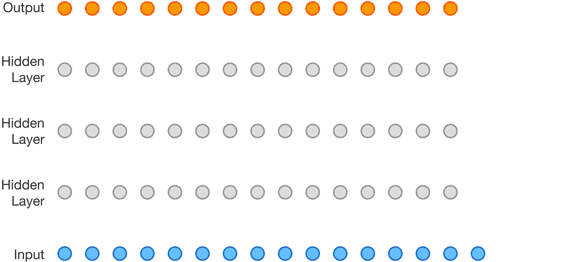​ Back to that equation real quick. The following equation should make some sense: $X_{t+1} = \sum^t_i \delta _i X_{t-i} + c$ but you are probably wondering how this concept is applied to deep learning. A deep autoregressive model is conceptually very similar but the equation needs to be adapted to deep learning to account for the network structure. Most deep learning autoregressive networks can be modeled by something similar to the following: $\Pi ^T_{t=1}P(X_{t+1}|X_t, X_{t-1},...,X_1, X_0)$ or in words, this is the distribution of the next value given all previous values in the sequence. This is just the probability chain rule, one of the most fundamental equations in statistics, but when paired with deep learning architecture, this equation can be highly expressive. Often you need a discrete value not a distribution so you can use a softmaxed activation function to correct for this. A structurally similar equation might look something like $X_{x+1} = softmax[\Pi ^T_{t=1}P(X_{t+1}|X_t, X_{t-1},...,X_1, X_0)]$. It’s important to note that we need to use causal convolution to ensure that no data is allowed to leak backward in time i.e. all predictions are made using only data from previous time steps, this is necessary to preserve the validity of the chain rule mentioned above. Both the traditional model and the deep learning probabilistic model use previous data to predict future data, but the deep learning model is more powerful for several reasons primarily its ability to deal with large amounts of high dimensionality data.The network architecture helps the chain rule represent complex data in such a way that structure prediction is possible. For example, when trained on an image dataset, the deep learning model allows the chain rule to implicitly represent not only the probability of each pixel but also the relation between them. ​ From here, it’s a normal supervised learning technique where the loss is correlated with the difference between the predicted output and the observed next item in the sequence. During training, the input is the observed (original training) data not the predicted data which is notably not autoregressive, but it allows for a very high level of parallelization which speeds up training by a factor of 5 or 10 or more. It’s only during inference when it’s important that the output is fed back in as input. Conceptually, that’s all you really need to know about autoregressive networks. The interesting part is in the special cases, applications, and oddly enough the implementation itself.

## Applications

​ First, applications. Research in autoregressive networks tends toward two large subject areas: image generation and sequence modeling with a particular emphasis on audio generation and text to speech.

​ PixelCNN is one of the best known autoregressive networks and it basically treats images as a sequence of pixel vectors, from left to right, top to bottom where each pixel location has an R(ed), G(reen), and B(lue) dimension. PixelCNN basically builds a picture, pixel by pixel, from all the pixels upward and left of the current position, as shown below. This is an example of structured prediction as mentioned above.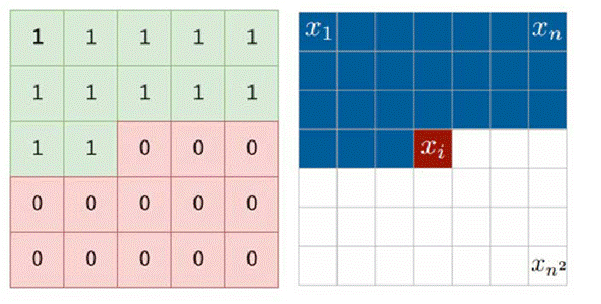Cited: https://www.codeproject.com/Articles/5061271/PixelCNN-in-Autoregressive-Models

​ If you only give it one pixel, it will try to generate something based on the training data but if you give it more information to condition on, maybe the top half of a picture, for example, it will attempt to complete the picture. Below is a collection of images finished by the network after being given about half of the image.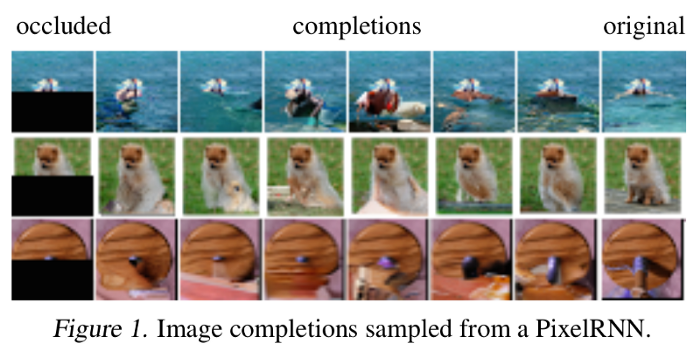Cited: https://arxiv.org/pdf/1606.05328.pdf

​ As you can see, it’s not exactly state of the art in terms of image generation, but conceptually, autoregressive models might offer better density estimation than GANs and possibly VAEs in addition to reduced inductive bias. Autoregressive models certainly have some distance to go in image generation but the potential is promising. (to web dev try to make this a caption or comment on the picture if possible)

​ The other large research area in autoregressive deep models is in sequence data because this is where autoregressive models truly excel. One of the best-known models in this field is WaveNet. The fact that autoregressive models are supervised sequential feed-forward models allows for more robust audio generation than almost any other model. As mentioned above, the fact that these networks can be effectively parallelized speeds up operations in both training and inference dramatically especially over traditional sequential models like RNNs. Normally, raw audio is incredibly hard to generate because of the sheer number of predictions required.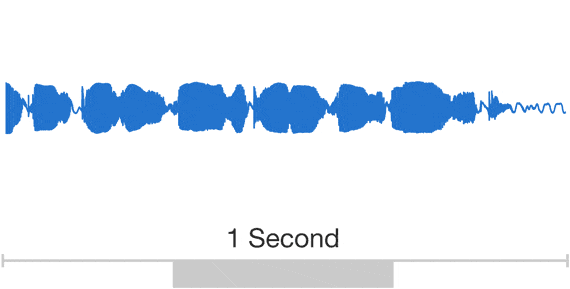The feed-forward nature of the network allows for precise, high-volume output; therefore requiring minimal interpolation as might be for RNNs or similar models. The fact that this ends up being a traditional supervised learning task is more of a practical advantage than a theoretical one. ​

​ Generating complex, structured data such as pictures and audio has been an important field of research recently, brought to the spotlight in part by photorealistic images generated using a GAN and later VAEs. These models made enormous contributions to the field but they also posed new challenges as they are unstable and unsupervised. Machine learning researchers have developed a very organized way to train, verify, and test normal supervised learning tasks, but high entropy generation via GANs, VAEs and others have not benefited from these methods as they are unsupervised. Autoregressive models are somewhat unique in a task dominated by unsupervised models, and this might provide benefits related to supervised tasks.

​ Autoregressive networks also avoid some of the common pitfalls of other sequence models by simply limiting complexity. Training RNNs is difficult for a number of reasons. One big issue with training RNNs is the optimization method: backpropagation through time. This particular method has notorious issues with getting stuck at poorly located local minima due to the recurrent feedback from the network, not present in normal feedforward networks. And of course, the vanishing / exploding gradient problem is of particular concern to recurrent networks. Autoregressive networks just don’t have this problem. The simplicity of the architecture avoids or at least mitigates many common training issues. But once again, no free lunch. Autoregressive models do have one caveat that makes them theoretically less powerful than RNNs. Autoregressive networks don’t have unlimited ‘memory’, the earliest piece of data an AR model can use is the first input in its receptive field and the receptive field is a sliding window. There’s no ‘memory’ functionality in autoregressive models which sounds like it would be a major issue, but recent research suggests that in practice, it’s much less of a handicap than might be assumed. The following excerpt from the article linked above nicely summarizes the issue: “Recurrent models trained in practice are effectively feed-forward. This could happen either because truncated backpropagation time cannot learn patterns significantly longer than K steps, or, more provocatively, because models trainable by gradient descent cannot have long-term memory.”

​ All of this suggests that recent AR models might be very promising. WaveNet is the current field leader in text to speech in terms of auditory similarity to human voice.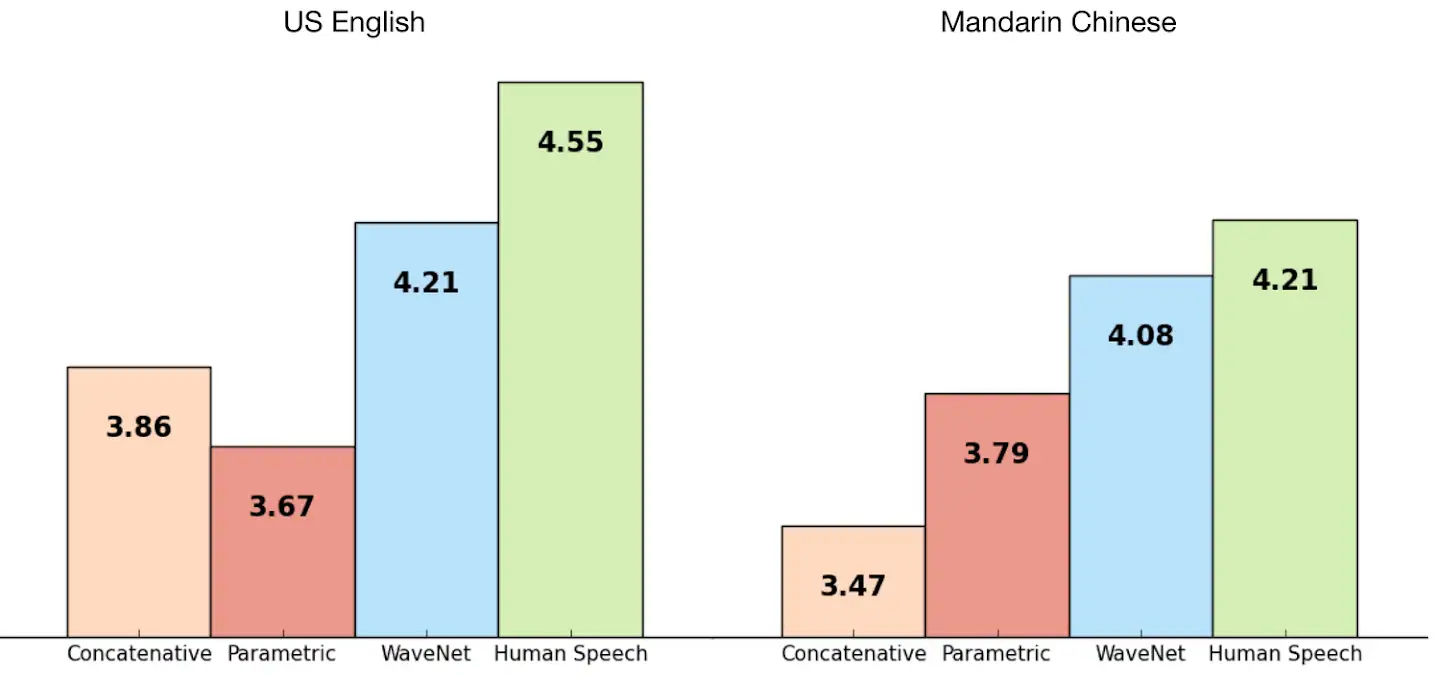Cited: https://deepmind.com/blog/article/wavenet-generative-model-raw-audio

​ Applying the generative and sequential properties to audio also leads to some interesting results. When trained on classical piano data, and completely unconditioned (well cover that next but it basically means they lat the model do what it wanted) WaveNet made this: https://storage.googleapis.com/deepmind-media/research/WaveNet/Music/sample_1.wavCited: https://deepmind.com/blog/article/wavenet-generative-model-raw-audio

## Conditioning

​ Once a model is trained, the next trick is getting it to say the word you want it to say. Keeping with the example of text to speech, you want the model to decide what actual sound is produced, but obviously the model needs to say what’s written, not what it wants. This is where conditioning comes in. Remember the equation from above? $X_{x+1} = softmax[\Pi ^T_{t=1}P(X_{t+1}|X_t, X_{t-1},...,X_1, X_0)]$ Currently, this says that the next output is dependant solely on all previous inputs. That doesn’t take into account the fact that it needs to say a specific thing, however we can fix this by simply conditioning on one more term.$X_{x+1} = softmax[\Pi ^T_{t=1}P(X_{t+1}|X_t, X_{t-1},...,X_1, X_0| Z)]$ Now the next output is dependant on both all* previous input and Z where Z is how we tell it what we want it to say. Normally, this is a simple function that relates the word to a unique value, basically one-hot encoding, but can be more complex functions for other applications. ​ This is a relatively simple structural change that allows for a lot of possibilities. The ability to select the next word being spoken or the class of picture being generated is obviously essential but we can also get more creative. Being able to guide the network makes it very easy to generate specific things. In audio, this might allows for the speaker’s voice to change or to make it sound sung instead of spoken. In pictures or videos, this usually accounts for the class that the model makes. (dog vs cat or whatever i gotta reword that) ​ Originally, researchers thought that autoregressive models could only be conditioned on the data’s labels, but a clever workaround fixed this. DeepMind basically made an autoencoder but replaced the decoder with PixelCNN and conditioned on the latent vector. This works both globally and locally. So on WaveNet for example, local conditioning might be used to make the model say a specific word, but global conditioning would be used to change the voice of the speaker. *not technically all, only the inputs in the receptive field

## Details

​ The main advantage of autoregressive models over other similar models is training speed and stability in exchange for complexity. Almost all increased performance in speed is due to the ability to effectively parallelize computations during training. This is massively advantageous on modern computers and specifically, GPUs which allow for many operations to be completed at the same time as long as the output from one isn’t necessary for the next. If this sounds directly in conflict with autoregression you have a point, however as I mentioned above, in training, the value the model generates isn’t actually fed back in as input, the actual value at that timestep is fed back in. Because we already know what the value is supposed to be at all time steps, all the calculations are independent and therefore parallelizable. This, however, does not work in inference, when generating models, the previous output is required to find the next. This means that autoregressive models are very fast in training but until recently, very slow in inference. This has since been somewhat overcome by caching hidden states to void recomputation which speeds up inference significantly. ​ The other main advantage over other generative models is stability. GAN’s have a notorious issue where they simply collapse and only spit out random noise, and VAEs, often have issues in the latent regularization step leading to some inconsistent results. AR models are stable and simple. They are traditional feedforward networks and work therefore don’t have stability issues or related ills. They also avoid the vanishing / exploding gradient problem because of the shortened path of backpropagation.

​ Autoregressive models offer an interesting tradeoff between speed, stability, and complexity that will continue to be explored in the near future, but for now, are state of the art sequence models with compelling applications to non-sequential tasks like image generation. Their simplicity allows for the application of decades of research in machine learning training but may also be their most limiting factor.